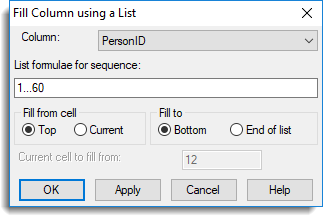1. Home
2. Fill Using a List

# Fill Using a List

List fill lets you specify a general patterned sequence of numbers to be entered into a column.

1. Select Spread | Calculate | List Fill.
2. From the dropdown list select the column you want to fill.3. Enter values as required then click Apply to fill the column and keep the dialog open.
4. To fill other columns repeat from step 2 then click OK when all required columns are filled.
 List formulae for sequence Specify a formula to generate a sequence of numbers to be inserted into the column. A list is a sequence of numbers separated by commas. The list can contain progressions and pre- or post-multipliers. A progression is a set of numbers ascending or descending with equal increments, and can be specified succinctly using the form “number, number…number” where the first two numbers define the first two elements in the list (and thus the increment) and the list ends with the value beyond which the third number would be passed. For lists with an increment of plus or minus one, the second number can be omitted, to give the form “number…number”. For example the progressions 1…5 = 1,2,3,4,5, 10…6 = 10,9,8,7,6 and 0,2…9 = 0,2,4,6,8 (this excludes 9, which is not in the sequence of adding the increment 2). A multiplier allows repetitive lists to be specified concisely. A pre-multiplier occurs immediately before the initial (round) bracket of a pair enclosing a list of numbers and has the effect of repeating each item in turn, the specified number of times. For example, 3(1,2) = 1,1,1,2,2,2. A post-multiplier is given immediately after the second of a pair of round brackets enclosing a list of numbers and has the effect of repeating the entire list as a whole, the specified number of times. For example, (1,2)3 = 1,2,1,2,1,2. The progressions and multipliers can be combined as shown in the examples below. 3(4.8,9.3) = 4.8,4.8,4.8,9.3,9.3,9.3 2(1…4) = 1,1,2,2,3,3,4,4 2(1,2,4)3 = 1,1,2,2,4,4,1,1,2,2,4,4,1,1,2,2,4,4 (2(1),4(0))2 = 1,1,0,0,0,0,1,1,0,0,0,0 (1…3)2,3(4,5) = 1,2,3,1,2,3,4,4,5,5 (5…1),(1…5) = 5,4,3,2,1,1,2,3,4,5 3(0,(1,2)2) = 0,0,0,1,2,1,2,1,2 (1,3…11)2 = 1,3,5,7,9,11,1,3,5,7,9,11 Fill from cell Top  – Fills from the first cell in the column. Current – Fills from the cell specified in Current cell to fill from. By default this is the cell that has the cursor focus. Fill to Bottom – Fills all the cells, recycling the list if needed. End of list – Fills the cells until the list runs out, then stops. Current cell to fill from Specify the starting cell for the sequence.
Updated on December 14, 2018